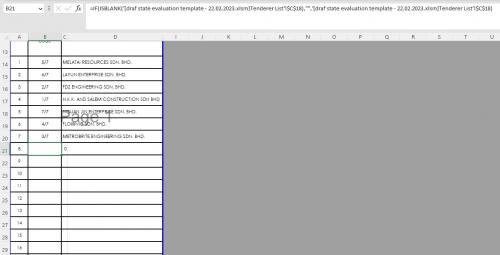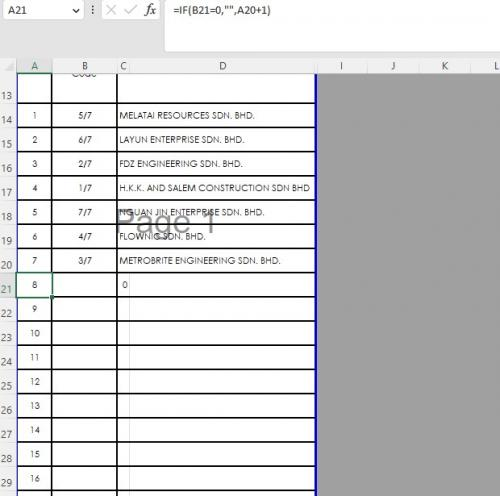Please Note: This article is written for users of the following Microsoft Excel versions: 2007, 2010, 2013, and 2016. If you are using an earlier version (Excel 2003 or earlier), this tip may not work for you. For a version of this tip written specifically for earlier versions of Excel, click here: Returning Zero when a Referenced Cell is Blank.

# Returning Zero When a Referenced Cell is BlankWritten by Allen Wyatt (last updated September 3, 2021)
This tip applies to Excel 2007, 2010, 2013, and 2016

If you have a formula in a worksheet, and the cell referenced by the formula is blank, then the formula still returns a zero value. For instance, if you have the formula =A3, then the formula returns the contents of cell A3, unless cell A3 is blank. In that case, the formula returns a value of zero.

This seems to be related to the idea that it is impossible for a formula to return a blank value, when "blank" is used synonymously with "empty." You can, however, expand your formula a bit so that it returns an empty string. Instead of using =A3 as your formula, you would use the following:

```=IF(ISBLANK(A3),"",A3)
```

This formula uses ISBLANK, which returns either True or False, depending on whether the referenced cell (A3) is blank or not. The IF function then returns an empty string ("") if A3 is blank, or it uses the value in A3 if A3 is not blank.

Regardless of what the formula returns, you can still use its result in other formulas, and it will work fine. Even if it returns an empty string, it is still treated by other formulas as if it contained zero. In areas where treating the cell as if it contained zero might be problematic (such as when you are charting the results of the formula), then you can modify the formula a bit, as shown here:

```=IF(ISBLANK(A3),NA(),A3)
```

This formula returns the #N/A error if A3 is blank. This error propagates through other formulas that reference the formula, but the #N/A error is ignored completely when charting.

While the above solutions are satisfactory for most people, some people would really like to see a target cell be truly blank if the source cell is blank. For instance, you might want cell B7 to be blank if cell A3 is blank. If you put a formula in cell B7 (as already discussed), then cell B7 is not truly blank—it contains a formula.

If this is your goal—true "blankness"—then you can only achieve it through the use of a macro. The macro will need to check to see if the source cell was changed. If it was, then whatever is in the source needs to be copied to the target cell.

```Private Sub Worksheet_Change(ByVal Target As Excel.Range)
Dim rMonitor As Range
Dim rTarget As Range

Set rMonitor = Range("A3")
Set rTarget = Range("B7")

If Not Intersect(Target, rMonitor) Is Nothing Then
rMonitor.Copy rTarget
End If

Set rMonitor = Nothing
Set rTarget = Nothing
End Sub
```

Note:

If you would like to know how to use the macros described on this page (or on any other page on the ExcelTips sites), I've prepared a special page that includes helpful information. Click here to open that special page in a new browser tab.

ExcelTips is your source for cost-effective Microsoft Excel training. This tip (10105) applies to Microsoft Excel 2007, 2010, 2013, and 2016. You can find a version of this tip for the older menu interface of Excel here: Returning Zero when a Referenced Cell is Blank.

##### Author Bio

Allen Wyatt

With more than 50 non-fiction books and numerous magazine articles to his credit, Allen Wyatt is an internationally recognized author. He is president of Sharon Parq Associates, a computer and publishing services company. ...

##### MORE FROM ALLEN

Setting Vertical Alignment

Excel allows you to adjust not only the horizontal alignment of values in a cell, but also the vertical alignment. This ...

Discover More

Missing Row Numbers

By default, Excel shows row numbers at the left side of your program window. If you cannot see the row numbers, there are ...

Discover More

Page Numbers in VBA

When you print a larger worksheet, Excel breaks the printout across several pages. You may want to know, before you ...

Discover MoreCreate Custom Apps with VBA! Discover how to extend the capabilities of Office 2013 (Word, Excel, PowerPoint, Outlook, and Access) with VBA programming, using it for writing macros, automating Office applications, and creating custom applications. Check out Mastering VBA for Office 2013 today!

##### More ExcelTips (ribbon)

Changing the Cycling Sequence for the F4 Cell Reference Shortcut

When editing a formula, the F4 shortcut key can be helpful. It may not, however, be helpful in all instances. This tip ...

Discover More

Relative References to Cells in Other Workbooks

When you construct a formula and click on a cell in a different workbook, an absolute reference to that cell is placed in ...

Discover More

Moving the House Number to Its Own Cell

Excel is great at manipulating data, but sometimes it is difficult to figure out the best way to do the manipulation. ...

Discover More
##### Subscribe

FREE SERVICE: Get tips like this every week in ExcelTips, a free productivity newsletter. Enter your address and click "Subscribe."

If you would like to add an image to your comment (not an avatar, but an image to help in making the point of your comment), include the characters [{fig}] (all 7 characters, in the sequence shown) in your comment text. You’ll be prompted to upload your image when you submit the comment. Maximum image size is 6Mpixels. Images larger than 600px wide or 1000px tall will be reduced. Up to three images may be included in a comment. All images are subject to review. Commenting privileges may be curtailed if inappropriate images are posted.

What is 2 + 2?

2023-03-03 10:59:24

J. Woolley

@Douglas
Perhaps B21 is not a blank cell but contains empty text like "" or a single apostrophe ('). Try this formula in cell A21:
=IF(OR(B21=0,B21=""),"",A20+1)

2023-03-02 22:13:38

Douglas

Hi, Allen.

i'm facing a problem whereby I have a cell (say; B21) which is referenced from another spreadsheet and I have keyed-in a formula to return it to a blank string. I would like the number sequence to follow suit whereby the blank cell it references (say; in cell A21, the formula is [if B21 = 0, "",B21+1]) if there is value in cell B21, then it returns a value but if cell B21 is blank, then it returns a blank in cell A21, but it returns a value to me even though the cell reference is blank. I tried doing running the macro on individual and ranged data but it still returns a value to me. Would really appreciate the help!

Attached here the formulas I keyed in (see Figure 1 below) (see Figure 2 below)Figure 1. Cell B21Figure 2. Cell A21

2021-09-03 13:10:49

David Bonin

I take a similar, though different, approach.

Most of my Excel work is for other people in a corporate environment -- often people I don't even know.

I never want to show an error value when a calculation doesn't have enough values to work correctly. Why? Because some users will assume the spreadsheet is broken.

I also never want to calculate an answer if the formula is missing some values. Why? Because some users will not notice the calculated value is not complete.

Here's an example... Suppose we put this formula in cell D1:
= ( A1 + B1 ) / C1

If C1 is blank or zero, we get an error.

If A1, B1 or both A1 and B1 are blank, we get a misleading answer.

Neither of these cases are good, so here's the alternate formula I would enter in D1:
= IF( COUNT( A1, B1, C1 ) = 3, (A1 + B1 ) / C1, "•" )

This formula will not give a numeric answer until A1, B1 and C1 all have values. The dot character is created by holding the Alt key down and typing 0149 (Alt+0149). I use the dot as it is unique and won't get confused with anything else. You can use whatever you like, such as "- -", "—", "n/a"...

Notice that I did not return a blank, ie: "". Why? Several reasons. Seeing a dot character tell me that there is indeed a formula in that cell and that I didn't accidentally delete it. On a large, complex spreadsheet, it's easy to accidentally delete something. (Ask me how I know.)

It also tells the user there is a formula in that cell and that he probably should not enter his own value there.

If there is a possibility the user might enter a zero in C1, then I might make the formula a bit more robust:
= IF( COUNT( A1, B1, C1 ) = 3, if( C1 <> 0, (A1 + B1 ) / C1, "Err: C1 = 0!" ), "•" )       << or >>
= IFERROR( IF( COUNT( A1, B1, C1 ) = 3, if( C1 <> 0, (A1 + B1 ) / C1, "•" ), "Err: C1 = 0!" )

You can put in whatever error message is most meaningful to your user.

To go a step further, an acre of dark dots can be distracting, so I usually apply a custom number format. If my usual number format would be "0.00;-0.00;0_._0_0;@", then I would use the similar "0.00;-0.00;0_._0_0;[COLOR16]@" so that the dots are light gray. Dots are still visible, but they're now unobtrusive.

There are other color codes available. Look them up. I use custom number formats instead of conditional formats when I can because custom number formats are more stable and they don't "breed".

(Back in my FORTRAN days, I always hated seeing a whole printed page of mostly 0.000 values with a few on-zero numbers sprinkled among them. I actually tweaked my formatting so that insignificant zeros just showed up as a decimal point " .    " to make them less distracting.)

2020-12-02 00:12:45

SteveF

I've been using Excel since the early 90's. This is a recent change in how Excel handles blank cells and NOT a welcome change. Until just recently, you could always expect to get a "" returned from an empty cell when referenced from another cell. Now they are converting the data type from the STRING you expect to a DOUBLE numeric of value 0. THIS IS COMPLETE BS and it is breaking thousands if not millions of older spreadsheets.

I don't understand any possible rationale for making this change to Excel. A completely misguided step backwards.

##### This Site

Got a version of Excel that uses the ribbon interface (Excel 2007 or later)? This site is for you! If you use an earlier version of Excel, visit our ExcelTips site focusing on the menu interface.